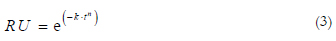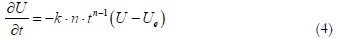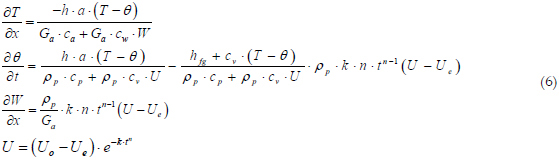# Abstracts

New formulation of the Michigan State University - MSU drying simulation model is proposed, based on Page’s thinlayer equation. It was derived, and the new drying rate formulation was used to define the humidity ratio of the air, and to complete the definition of the product temperature. All these resulted in less derivatives approximated by finite differences. The improved MSU model led to precise results, with large flexibility in time and space increments when simulation is made through finite differences, arriving to the solutions in less time, due to less derivatives numerically approximated.

coherent model formulation; coherent results; precise results

Nova formulação do modelo de secagem de Michigan é apresentada, com base na equação de secagem em camadas finas proposta por Page. Esta equação foi derivada e a nova taxa de secagem foi usada para definir a umidade absoluta do ar, assim como para completar a definição da variação da temperatura do produto. O uso dessa nova definição de taxa de secagem resultou em um sistema de equações sem nenhuma derivada nas definições das variáveis, o que resultou em menos equações derivadas aproximadas numericamente. Esse novo modelo de Michigan leva a resultados precisos, com grande flexibilidade em incrementos de tempo e de espaço quando resolvido por métodos numéricos, levando a soluções em menos tempo, pelo menor número de equações derivadas que precisaram ser aproximadas.

ENGENHARIA RURAL / AGRICULTURAL ENGINEERING

Performance of the Michigan drying simulation model with a new drying rate concept

Valdecir Antoninho DalpasqualeI* * Autor para correspondência. Email: valdecir.dalpasquale@gmail.com ; Décio SperandioII; Evandro Marcos KollingIII

IDepartamento de Agronomia, Centro de Ciências Agrárias, Universidade Estadual de Maringá, Av. Colombo, 5790, 87020900, Maringá, Paraná, Brasil

IIICentro de Educação Profissional de Cascavel, Cascavel, Paraná, Brasil

ABSTRACT

New formulation of the Michigan State University – MSU drying simulation model is proposed, based on Page’s thinlayer equation. It was derived, and the new drying rate formulation was used to define the humidity ratio of the air, and to complete the definition of the product temperature. All these resulted in less derivatives approximated by finite differences. The improved MSU model led to precise results, with large flexibility in time and space increments when simulation is made through finite differences, arriving to the solutions in less time, due to less derivatives numerically approximated.

Keywords: coherent model formulation, coherent results, precise results

RESUMO

Desempenho do modelo de simulação de secagem de Michigan com o novo conceito de taxa de secagem. Nova formulação do modelo de secagem de Michigan é apresentada, com base na equação de secagem em camadas finas proposta por Page. Esta equação foi derivada e a nova taxa de secagem foi usada para definir a umidade absoluta do ar, assim como para completar a definição da variação da temperatura do produto. O uso dessa nova definição de taxa de secagem resultou em um sistema de equações sem nenhuma derivada nas definições das variáveis, o que resultou em menos equações derivadas aproximadas numericamente. Esse novo modelo de Michigan leva a resultados precisos, com grande flexibilidade em incrementos de tempo e de espaço quando resolvido por métodos numéricos, levando a soluções em menos tempo, pelo menor número de equações derivadas que precisaram ser aproximadas.

Introduction

Drying of agricultural products can be understood as a process where moisture content is reduced to levels that allow long and safe preservation, using economical techniques of evaporation (DALPASQUALE, 2002). Drying can also be considered as a simultaneous heat and mass transfer process between the drying air and the product. Several authors have been publishing mathematical models on the drying process (SANDER et al., 2009; ARABHOSSEINI et al., 2009; ISLAM et al., 2005; PRINTE et al., 1995), although mathematically the drying concept was initially presented by Hukill (1954) apud Brooker et al. (1992), based on Newton's cooling law. The drying rate of an agricultural product, in an atmosphere with constant temperature, is proportional to the difference between product moisture content, U, and its equilibrium moisture, Ue, which can be expressed as:

The solution of equation 1 has exponential format:Equation 2 became known in the form RU = e(-k.t), where RU is the product dimensionless moisture ratio, and k a proportionality coefficient for each product. This formulation was successfully used until the popularization of the computer, when low precise values of the estimated moisture were observed, especially for air with high relative humidity.The parameters k and n were identified for different agricultural products (ASABE, 2009), and their use led to more accurate moisture content evaluations.

Hukill (1954) apud Brooker et al. (1992), started from the definition of drying rate to arrive to an analytical solution that estimates the moisture content of a product at different times. On the other hand, Sperandio (2005), starting from equation 3, arrived to its definition of drying rate. The new and more complete definition of drying rate is:Page (1949) apud Brooker et al. (1992), showed that improved moisture content estimates can be obtained if the solution of the drying rate equation would be expressed in the following way:

Equation 4 can be read as the drying rate of an agricultural product, in an atmosphere with constant temperature, decreases with the difference between product moisture content, U, and its equilibrium moisture, Ue, along with time. This new formulation is more coherent with the description of moisture removal of agricultural products. As drying progresses in products with high moisture contents, water is mainly removed from the capillary tubes. Next, the capillary tubes are destroyed and, soon afterwards, the removal of moisture concentrates in the dry matter particles. The moisture retention forces increase with the reduction of the available moisture. It is known that drying is more difficult as moisture is removed, which occurs as the time progresses. That is expressed in a more complete way by equation 4, which indicates explicitly the dependence of the drying rate also on the time spent for moisture removal, differently than equation 1.Among the mathematical models for simulation of drying of agricultural products, the Michigan State University  MSU model is among the most complete and better accepted. Drying in fixed-beds is expressed by the system of partial differential equations 5 (BROOKER et al., 1992).The last differential equation of the system is substituted, most of the time, for a thin-layer equation that evaluates the product moisture content at desirable times. Lately, equation 3 has been preferred for such a function.

Mathematical drying simulation is usually made taking care so the relative humidity of the air does not exceed 100%, with no reference to the enthalpy of the air (SPERANDIO, 2005), although it is widely accepted that the air behaves adiabatically in an agricultural drying process.

Drying simulation by computer has been faster than never, due to constant software technologies improvements, which does not guarantee improved accuracy in the results. Better accuracy was investigated in this work, not through numerical analysis arguments, but through more precise definition of drying rate. The objective of this work was to incorporate the new drying rate in the MSU fixed-bed drying model, and to compare its performance in regard to the original model.

Material and methods

In this work, Pages equation was used as the analytical solution of the fourth partial differential equation in the system of equations 5. For coherence, equation 4 was used in the definition of the third differential equation of the same system, and this new definition of the humidity ratio of the air, in the second partial differential equation of the system in analysis. This new formulation of the MSU fixed-bed drying simulation model (MSU6), along with the original one (MSU5), were solved using the methodology of finite differences, and the Newton Raphson's method to solve nonlinear algebraic systems that results in the application of the implicit/explicit finite difference method, which eliminates restrictions for time intervals (DALPASQUALE et al., 2007). Their results were compared to experimental corn drying conducted in a 0.8 m deep fixed-beds, originally with 18, 25 and 35% initial moisture contents, and dried at 40, 70 and 100ºC, respectively (PORTELLA, 2001), using space and time increments appropriated to the accuracy of the results.

Comparisons between these two models were also made by the enthalpy obtained in different points of the layer, using the three previous mentioned drying conditions. For all these tests, the amount of air used was 0.75 m3 s-1, from an environment of 22ºC and 60% relative humidity (PORTELLA, 2001).

Results and discussion

MSU6, the new formulation of the MSU fixedbed drying simulation model, coherent with the new definition of drying rate, acquires the form of equation 6.

This new description of the model of Michigan becomes dependent on the precision criteria of numerical analysis only at the left sides of the equations: drying air temperature, product temperature and humidity ratio of the air. The product moisture content is evaluated by Pages equation, equation 3, which is the solution of the new drying rate differential equation, equation 4. The variation of the humidity ratio of the air incorporated the new definition of drying rate, and this new definition of humidity ratio is used in the definition of product temperature, in the second equation of the system of partial differential equations 6

Experimental and simulated results are in Table 1, along with results simulated by MSU5 and MSU6. The used time interval in MSU5 was of a twentieth of the total drying time, although much lower values were recommended when different mathematical tools were used (BROOKER et al., 1992). Similar approach was employed for space increment, which was also of a twentieth of the thickness of the layer. These time and space increments allowed to simulate the experimental moisture content values, but with some differences in the drying time. The greater the drying rate, the larger the drying time differences between simulated and experimental results, when using MSU5.

MSU6 had a better performance. Even with time and space increments four times larger than those of the previous model, the results matched in moisture content and drying time, as shown in Table 1. The only difference was of 0.17 of an hour less simulation time for corn with 35% initial moisture content, dried at 100ºC, reassuring the better performance of MSU6 in regard to MSU5.Enthalpy values were obtained for the two MSU drying simulation models. In theory, those values should be the same, or without significant differences. In Tables 2 and 3, enthalpy values are presented for the air entrance in the drying layer and for the exit condition, at every one fifth of the drying time. Table 2 shows that, as the pair of corn initial moisture content/temperature of the drying air increases, increases the variation of enthalpy, differently than it should be in a process of agricultural product drying. The results in Table 2 show some variation among the enthalpies at the entrance and those at the exit, which indicate certain instability in the simulated results. In the results for corn initial moisture content of 35% and drying temperature of 100ºC, that instability happens in the first point of the exit enthalpy, and the differences among the entrance enthalpies and those of exit were larger than for the two previous groups of results. This should be due to certain incoherence among the equations of the system 5, as well as to the significant numerical dependence of the results.

Table 3

The coherence in the formulation of the system of partial differential equations 6, when solved by methods of finite differences became numerically more stable than the system of equations 5, without demanding any refined mesh. As a consequence, it is possible to simulate experimental values of drying with larger increments of time and space, which reduces the time for the solution of the system of equations. If this is not a major problem for the actual computing systems, it is always a difficulty in the identification of time and space steps for the proper accuracy, when standard numerical methods are used. This inference explains the same moisture predicted by MSU6 in Table 1, when a larger mesh than MSU5 was used for drying simulation, as well as the better predicted enthalpy results of Table 3.

Conclusion

The system of partial differential equations 6 is coherent in its formulation, due to a new definition of drying rate based on Pages equation for solution (SPERANDIO, 2005).

The Pages equation, like the new definition of drying rate, was incorporated into the Michigan drying simulation model. Because of those arrangements, the three equations of system 6 that depend directly or indirectly on the variation of the product moisture content do not do numerical approaches on their right sides to quantify the properties, because there are no derivatives on that side of the formulation of the model, what turns the solution more precise.

Symbols

A: particle surface area per unit volume (m2 m-3);

ca: specific heat of the air (J kg-1 ºC-1);

cp: specific heat of dry product kernels (J kg-1 ºC-1);

cv: specific heat water vapor (J kg-1 ºC-1);

cw: specific heat of liquid water (J kg-1 ºC-1);

Ga: airflow rate (kgd.a. hr-1 m-2);

h: convective heat transfer coefficient (J m-2 ºC-1 hr-1);

hfg: heat of evaporation (J kg-1);

k: drying constant (hr-1);

n: time exponent;

t: drying time (hr);

T: air temperature (ºC);

U: product moisture content (dry basis);

Ue: product equilibrium moisture content (dry basis);

W: air humidity ratio (kgw gd.a.-1);

x: bed coordinate (m);

ρp: product density (kg dry product m-3).

Accepted on February 18, 2009.

License information: This is an openaccess article distributed under the terms of the Creative Commons Attribution License, which permits unrestricted use, distribution, and reproduction in any medium, provided the original work is properly cited.

• ASABE-American Society of Agricultural and Biological Engineering. Standards. St. Joseph, 2009.
• ARABHOSSEINI, A.; HUISMAN, W.; VAN BOXTEL, A.; MULLER, J. Modeling of thin layer drying of tarragon (Artemisia dracunculus L.). Industrial Crops and Products, v. 29, n. 1, p. 53-59, 2009.
• BROOKER, D. B.; BAKKER-ARKEMA, F. W.; HALL C. W. Drying and Storage of Grains and Oilseeds. New York: An AVI Book, 1992.
• DALPASQUALE, V. A.; SPERANDIO, D.; MONKEN E SILVA, L. H.; KOLLING, E. Fixed-bed drying simulation of agricultural products using a new backward finite difference scheme. Applied Mathematics and Computation, v. 25, n. 2, p. 675-684, 2007.
• ISLAM, M.T.; MARKS, B.P.; BAKKER-ARKEMA, F. W. Modeling an ear-corn dryer. Transactions of the Asae, v. 48, n. 1, p. 243-249, 2005.
• PORTELLA, J. A. Secagem de grãos. 1. ed. Passo Fundo: Embrapa Trigo, 2001.
• PRIANTE, N.; DEMUSIS, C. R.; CANEPPELE, C.; CAMPELO, J. H.; SADHU, D. Simulation of grain drying in natural-convection dryer. Drying Tecnhology, v. 13, n. 1-2, p. 165-181, 1995.
• SANDER, A.; KARDUM, J. P. Experimental validation of thin-layer drying models Chemical Engineering and Technology, v. 32, n. 4, p. 590-599, 2009.
• SPERANDIO, D. Secagem de produtos agrícolas em camadas fixas e em fluxos cruzados sob o efeito de entalpia constante. 2005. Tese (Doutorado) - Universidade Estadual de Maringá, Maringá, 2005.
• *
Autor para correspondência. Email:

# Publication Dates

• Publication in this collection
25 Aug 2011
• Date of issue
Dec 2009

• Accepted
18 Feb 2009